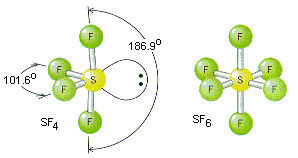The Chemistry of Oxygen and Sulfur

The Chemistry of Oxygen and Sulfur

The Chemistry of Oxygen

Oxygen is the most abundant element on this planet. The earth's crust is 46.6% oxygen by weight, the oceans are 86% oxygen by weight, and the atmosphere is 21% oxygen by volume. The name oxygen comes from the Greek stems oxys, "acid," and gennan, "to form or generate." Thus, oxygen literally means "acid former." This name was introduced by Lavoisier, who noticed that compounds rich in oxygen, such as SO2 and P4O10, dissolve in water to give acids.

The electron configuration of an oxygen atom[He] 2s2 2p4suggests that neutral oxygen atoms can achieve an octet of valence electrons by sharing two pairs of electrons to form an O=O double bond, as shown in the figure below.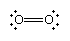According to this Lewis structure, all of the electrons in the O2 molecule are paired. The compound should therefore be diamagneticit should be repelled by a magnetic field. Experimentally, O2 is found to be paramagneticit is attracted to a magnetic field. This can be explained by assuming that there are two unpaired electrons in the* antibonding molecular orbitals of the O2 molecule.This photograph shows that the liquid O2 is so strongly attracted to a magnetic field that it will bridge the gap between the poles of a horseshoe magnet.

At temperatures below -183oC, O2 condenses to form a liquid with a characteristic light blue color that results from the absorption of light with a wavelength of 630 nm. This absorption is not seen in the gas phase and is relatively weak even in the liquid because it requires that three bodiestwo O2 molecules and a photoncollide simultaneously, which is a very rare phenomenon, even in the liquid phase.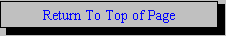The Chemistry of Ozone

The O2 molecule is not the only elemental form of oxygen. In the presence of lightning or another source of a spark, O2 molecules dissociate to form oxygen atoms.

 spark O2(g)2 O(g)

These O atoms can react with O2 molecules to form ozone, O3,

 O2(g) + O(g)O3(g)

whose Lewis structure is shown in the figure below.Oxygen (O2) and ozone (O3) are examples of allotropes (from the Greek meaning "in another manner"). By definition, allotropes are different forms of an element. Because they have different structures, allotropes have different chemical and physical properties (see table below).

Properties of Allotropes of Oxygen

 Oxygen (O2 ) Ozone (O3 ) Melting Point -218.75oC -192.5oC Boiling Point -182.96oC -110.5oC Density (at 20oC) 1.331 g/L 1.998 g/L O-O bond order 2 1.5 O-O bond length 0.1207 nm 0.1278 nm

Ozone is an unstable compound with a sharp, pungent odor that slowly decomposes to oxygen.

 3 O3(g)3 O2(g)

At low concentrations, ozone can be relatively pleasant. (The characteristic clean odor associated with summer thunderstorms is due to the formation of small amounts of O3.)

Exposure to O3 at higher concentrations leads to coughing, rapid beating of the heart, chest pain, and general body pain. At concentrations above 1 ppm, ozone is toxic.

One of the characteristic properties of ozone is its ability to absorb radiation in the ultraviolet portion of the spectrum ( > 300 nm), thereby providing a filter that protects us from exposure to high-energy ultraviolet radiation emitted by the sun. We can understand the importance of this filter if we think about what happens when radiation from the sun is absorbed by our skin.

Electromagnetic radiation in the infrared, visible, and low-energy portions of the ultraviolet spectrum ( < 300 nm) carries enough energy to excite an electron in a molecule into a higher energy orbital. This electron eventually falls back into the orbital from which it was excited and energy is given off to the surrounding tissue in the form of heat. Anyone who has suffered from a sunburn can appreciate the painful consequences of excessive amounts of this radiation.

Radiation in the high-energy portion of the ultraviolet spectrum ( 300 nm) has a different effect when it is absorbed. This radiation carries enough energy to ionize atoms or molecules. The ions formed in these reactions have an odd number of electrons and are extremely reactive. They can cause permanent damage to the cell tissue and induce processes that eventually result in skin cancer. Relatively small amounts of this radiation can therefore have drastic effects on living tissue.

In 1974 Molina and Rowland pointed out that chlorofluorocarbons, such as CFCl3 and CF2Cl2, which had been used as refrigerants and as propellants in aerosol cans, were beginning to accumulate in the atmosphere. In the stratosphere, at altitudes of 10 to 50 km above the earth's surface, chlorofluorocarbons decompose to form Cl atoms and chlorine oxides such as ClO when they absorb sunlight. Cl atoms and ClO molecules have an odd number of electrons, as shown in the figure below.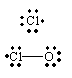As a result, these substances are unusually reactive. In the atmosphere, they react with ozone or with the oxygen atoms that are needed to form ozone.

 Cl + O3ClO + O2 ClO + OCl + O2

Molina and Rowland postulated that these substances would eventually deplete the ozone shield in the stratosphere, with dangerous implications for biological systems that would be exposed to increased levels of high-energy ultraviolet radiation.Oxygen as an Oxidizing Agent

Fluorine is the only element that is more electronegative than oxygen. As a result, oxygen gains electrons in virtually all its chemical reactions. Each O2 molecule must gain four electrons to satisfy the octets of the two oxygen atoms without sharing electrons, as shown in the figure below.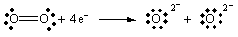Oxygen therefore oxidizes metals to form salts in which the oxygen atoms are formally present as O2- ions. Rust forms, for example, when iron reacts with oxygen in the presence of water to give a salt that formally contains the Fe3+ and O2- ions, with an average of three water molecules coordinated to each Fe3+ ions in this solid.

 H2O 4 Fe(s) + 3 O2(g)2 Fe2O3(s) 3 H2O

Oxygen also oxidizes nonmetals, such as carbon, to form covalent compounds in which the oxygen formally has an oxidation number of -2.

 C(s) + O2(g)CO2(g)

Oxygen is the perfect example of an oxidizing agent because it increases the oxidation state of almost any substance with which it reacts. In the course of its reactions, oxygen is reduced. The substances it reacts with are therefore reducing agents.Peroxides

It takes four electrons to reduce an O2 molecule to a pair of O2- ions. If the reaction stops after the O2 molecule has gained only two electrons, the O22- ion shown in the figure below is produced.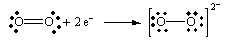This ion has two more electrons than a neutral O2 molecule, which means that the oxygen atoms must share only a single pair of bonding electrons to achieve an octet of valence electrons. The O22- ion is called the peroxide ion because compounds that contain this ion are unusually rich in oxygen. They are not just oxidesthey are (hy-)peroxides.

The easiest way to prepare a peroxide is to react sodium or barium metal with oxygen.

 2 Na(s) + O2(g)Na2O2(s) Ba(s) + O2(g)BaO2(s)

When these peroxides are allowed to react with a strong acid, hydrogen peroxide (H2O2) is produced.

 BaO2(s) + 2 H+(aq)Ba2+(aq) + H2O2(aq)

The Lewis structure of hydrogen peroxide contains an O-O single bond, as shown in the figure below.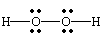The VSEPR theory predicts that the geometry around each oxygen atom in H2O2 should be bent. But this theory cannot predict whether the four atoms should lie in the same plane or whether the molecule should be visualized as lying in two intersecting planes. The experimentally determined structure of H2O2 is shown in the figure below.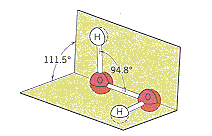The H-O-O bond angle in this molecule is only slightly larger than the angle between a pair of adjacent 2p atomic orbitals on the oxygen atom, and the angle between the planes that form the molecule is slightly larger than the tetrahedral angle.

The oxidation number of the oxygen atoms in hydrogen peroxide is -1. H2O2 can therefore act as an oxidizing agent and capture two more electrons to form a pair of hydroxide ions, in which the oxygen has an oxidation number of -2.

 H2O2 + 2 e-2 OH-

Or, it can act as a reducing agent and lose a pair of electrons to form an O2 molecule.

 H2O2O2 + 2 H+ + 2 e-

Reactions in which a compound simultaneously undergoes both oxidation and reduction are called disproportionation reactions. The products of the disproportionation of H2O2 are oxygen and water.

 2 H2O2(aq)O2(g) + 2 H2O(l)

The disproportionation of H2O2 is an exothermic reaction.

 2 H2O2(aq)O2(g) + 2 H2O(l)Ho = -94.6 kJ/mol H2O

This reaction is relatively slow, however, in the absence of a catalyst, such as dust or a metal surface. The principal uses of H2O2 revolve around its oxidizing ability. It is used in dilute (3%) solutions as a disinfectant. In more concentrated solutions (30%), it is used as a bleaching agent for hair, fur, leather, or the wood pulp used to make paper. In very concentrated solutions, H2O2 has been used as rocket fuel because of the ease with which it decomposes to give O2.Methods of Preparing O2

Small quantities of O2 gas can be prepared in a number of ways.

1. By decomposing a dilute solution of hydrogen peroxide with dust or a metal surface as the catalyst.

 2 H2O2(aq)O2(g) + 2 H2O(l)

2. By reacting hydrogen peroxide with a strong oxidizing agent, such as the permanganate ion, MnO4-.

 5 H2O2(aq) + 2 MnO4-(aq) + 6 H+(aq)2 Mn2+(aq) + 5 O2(g) + 8 H2O(l)

3. By passing an electric current through water.

 electrolysis 2 H2O(l)2 H2(g) + O2(g)

4. By heating potassium chlorate (KClO3) in the presence of a catalyst until it decomposes.

 MnO2 2 KClO3(s)2 KCl(s) + 3 O2(g)The Chemistry of Sulfur

Because sulfur is directly below oxygen in the periodic table, these elements have similar electron configurations. As a result, sulfur forms many compounds that are analogs of oxygen compounds, as shown in the table below. Examples in this table show how the prefix thio- can be used to indicate compounds in which sulfur replaces an oxygen atom. The thiocyanate (SCN-) ion, for instance, is the sulfur-containing analog of the cyanate (OCN-) ion.

Oxygen Compounds and Their Sulfur Analogs

 Oxygen Compounds Sulfur Compounds Na2O (sodium oxide) Na2S (sodium sulfide) H2O (water) H2S (hydrogen sulfide) O3 (ozone) SO2 (sulfur dioxide) CO2 (carbon dioxide) CS2 (carbon disulfide) OCN- (cyanate) SCN- (thiocyanate) OC(NH2)2 (urea) SC(NH2)2 (thiourea)

There are four principal differences between the chemistry of sulfur and oxygen.

1. O=O double bonds are much stronger than S=S double bonds.

2. S-S single bonds are almost twice as strong as O-O single bonds.

3. Sulfur (EN = 2.58) is much less electronegative than oxygen (EN = 3.44).

4. Sulfur can expand its valence shell to hold more than eight electrons, but oxygen cannot.

These seemingly minor differences have important consequences for the chemistry of these elements.The Effect of Differences in the Strength of X-X and X=X Bonds

The radius of a sulfur atom is about 60% larger than that of an oxygen atom.As a result, it is harder for sulfur atoms to come close enough together to form bonds. S=S double bonds are therefore much weaker than O=O double bonds.

Double bonds between sulfur and oxygen or carbon atoms can be found in compounds such as SO2 and CS2 (see figure below). But these double bonds are much weaker than the equivalent double bonds to oxygen atoms in O3 or CO2. The bond dissociation enthalpy for a C=S double bond is 477 kJ/mol, for example, whereas the bond dissociation enthalpy for a C=O double bond is 745 kJ/mol.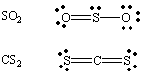Elemental oxygen consists of O2 molecules in which each atom completes its octet of valence electrons by sharing two pairs of electrons with a single neighboring atom. Because sulfur does not form strong S=S double bonds, elemental sulfur usually consists of cyclic S8 molecules in which each atom completes its octet by forming single bonds to two neighboring atoms, as shown in the figure below.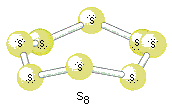S8 molecules can pack to form more than one crystal. The most stable form of sulfur consists of orthorhombic crystals of S8 molecules, which are often found near volcanoes. If these crystals are heated until they melt and the molten sulfur is then cooled, an allotrope of sulfur consisting of monoclinic crystals of S8 molecules is formed. These monoclinic crystals slowly transform themselves into the more stable orthorhombic structure over a period of time.

The tendency of an element to form bonds to itself is called catenation (from the Latin catena, "chain"). Because sulfur forms unusually strong S-S single bonds, it is better at catenation than any element except carbon. As a result, the orthorhombic and monoclinic forms of sulfur are not the only allotropes of the element. Allotropes of sulfur also exist that differ in the size of the molecules that form the crystal. Cyclic molecules that contain 6, 7, 8, 10, and 12 sulfur atoms are known.

Sulfur melts at 119.25oC to form a yellow liquid that is less viscous than water. If this liquid is heated to 159oC, it turns into a dark red liquid that cannot be poured from its container. The viscosity of this dark red liquid is 2000 times greater than that of molten sulfur because the cyclic S8 molecules open up and link together to form long chains of as many as 100,000 sulfur atoms.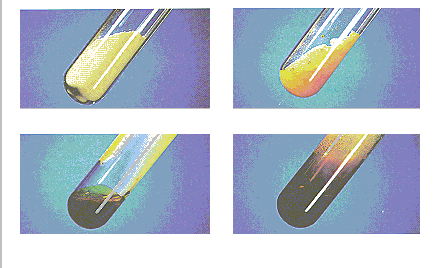When sulfur reacts with an active metal, it can form the sulfide ion, S2-.

 16 K(s) + S8(s)8 K2S(s)

This is not the only product that can be obtained, however. A variety of polysulfide ions with a charge of -2 can be produced that differ in the number of sulfur atoms in the chain.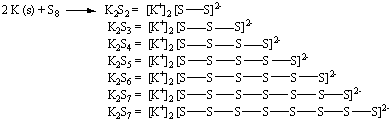The Effect of Differences in the Electronegativities of Sulfur and Oxygen

Because sulfur is much less electronegative than oxygen, it is more likely to form compounds in which it has a positive oxidation number (see table below).

Common Oxidation Numbers for Sulfur

 Oxidation Number Examples -2 Na2S, H2S -1 Na2S2, H2S2 0 S8 +1 S2Cl2 +2 S2O32- +21/2 S4O62- +3 S2O42- +4 SF4, SO2, H2SO3, SO32- +5 S2O62- +6 SF6, SO3, H2SO4, SO42-

In theory, sulfur can react with oxygen to form either SO2 or SO3, whose Lewis structures are given in the figure below.

 SO2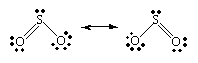SO3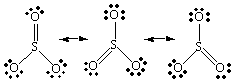In practice, combustion of sulfur compounds gives SO2, regardless of whether sulfur or a compound of sulfur is burned.

 S8(s) + 8 O2(g)8 SO2(g) CS2(l) + 3 O2(g)CO2(g) + 2 SO2(g) 3 FeS2(s) + 8 O2(g)Fe3O4(s) + 6 SO2(g)

Although the SO2 formed in these reactions should react with O2 to form SO3, the rate of this reaction is very slow. The rate of the conversion of SO2 into SO3 can be greatly increased by adding an appropriate catalyst.

 V2O5/K2O 2 SO2(g)2 SO3(g)

Enormous quantities of SO2 are produced by industry each year and then converted to SO3, which can be used to produce sulfuric acid, H2SO4. In theory, sulfuric acid can be made by dissolving SO3 gas in water.

 SO3(g) + H2O(l)H2SO4(aq)

In practice, this is not convenient. Instead, SO3 is absorbed in 98% H2SO4, where it reacts with the water to form additional H2SO4 molecules. Water is then added, as needed, to keep the concentration of this solution between 96% and 98% H2SO4 by weight.

Sulfuric acid is by far the most important industrial chemical. It has even been argued that there is a direct relationship between the amount of sulfuric acid a country consumes and its standard of living. More than 50% of the sulfuric acid produced each year is used to make fertilizers. The rest is used to make paper, synthetic fibers and textiles, insecticides, detergents, feed additives, dyes, drugs, antifreeze, paints and enamels, linoleum, synthetic rubber, printing inks, cellophane, photographic film, explosives, automobile batteries, and metals such as magnesium, aluminum, iron, and steel.

Sulfuric acid dissociates in water to give the HSO4- ion, which is known as the hydrogen sulfate, or bisulfate, ion.

 H2SO4(aq)H+(aq) + HSO4-(aq)

10% of these hydrogen sulfate ions dissociate further to give the SO42-, or sulfate, ion.

 HSO4-(aq)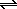H+(aq) + SO42-(aq)

A variety of salts can be formed by replacing the H+ ions in sulfuric acid with positively charged ions, such as the Na+ or K+ ions.

 NaHSO4 = sodium hydrogen sulfate Na2SO4 = sodium sulfate

Sulfur dioxide dissolves in water to form sulfurous acid.

 SO2(g) + H2O(l)H2SO3(aq)

Sulfurous acid doesn't dissociate in water to as great extent as sulfuric acid, but it is still possible to replace the H+ ions in H2SO3 with positive ions to form salts.

 NaHSO3 = sodium hydrogen sulfite Na2SO3 = sodium sulfite

Sulfuric acid and sulfurous acid are both examples of a class of compounds known as oxyacids, because they are literally acids that contain oxygen. Because they are negative ions (or anions) that contain oxygen, the SO32- and SO42- ions are known as oxyanions. The Lewis structures of some of the oxides of sulfur that form oxyacids or oxyanions are given in the table below.

 OXYACIDS OXYANIONS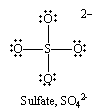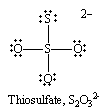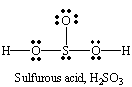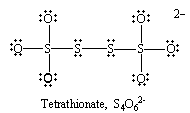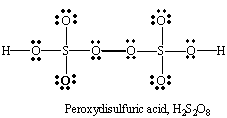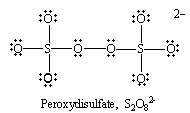One of these oxyanions deserves special mention. This ion, which is known as the thiosulfate ion, is formed by the reaction between sulfur and the sulfite (SO32-) ion.

 8 SO32-(aq) + S8(s)8 S2O32-(aq)The Effect of Differences in the Abilities of Sulfur and Oxygen to Expand Their Valence Shell

The electron configurations of oxygen and sulfur are usually written as follows.

 O = [He] 2s2 2p4 S = [Ne] 3s2 3p4

Although this notation shows the similarity between the configurations of the two elements, it hides an important difference that allows sulfur to expand its valence shell to hold more than eight electrons.

Oxygen reacts with fluorine to form OF2.

 O2(g) + 2 F2(g)2 OF2(g)

The reaction stops at this point because oxygen can hold only eight electrons in its valence shell, as shown in the figure below.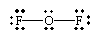Sulfur reacts with fluorine to form SF4 and SF6, shown in the figure below, because sulfur can expand its valence shell to hold 10 or even 12 electrons.

 S8(s) + 16 F2(g)8 SF4(g) S8(s) + 24 F2(g)8 SF6(g)